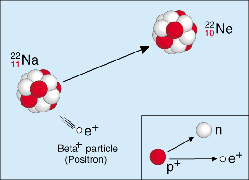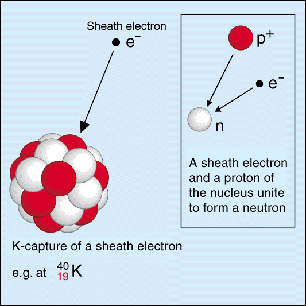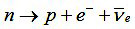A# beta decayFigure 1. Beta-minus decay.Figure 2. Beta-plus decay.Figure 3. Electron capture.

Beta decay is radioactive decay (see radioactivity) by emission of a beta particle. This process proceeds via the weak interaction and includes all nuclear decays in which the mass number, A, remains constant and the atomic number, Z, changes by one unit.

There are three main types of beta decay.

## Beta-minus decay

Nuclei that are rich in neutrons tend to decay by emitting an electron along with an antineutrino. As a result, one of the neutrons in the nucleus changes into a proton. E.g., decay of P-32 into S-32 or Cs-137 into Ba-137. See Figure 1.

## Beta-plus decay

Neutron-deficient nuclei tend to decay by positron emission or electron capture (see below). Positron emission refers to the emission of a positron along with a neutrino and the resulting conversion of a proton inside the nucleus into a neutron. E.g., decay of Na-22 into Ne-22. See Figure 2.

## Electron capture

Electron capture is usually classified as a type of beta decay and involves an orbital electron being absorbed by a nucleus, effectively converting a proton into a neutron. E.g., K-40, Mn-54, and Fe-55 radionuclides decay by this process. See Figure 3.

These three types of beta decay can be summarized as follows:The simplest beta decay process is free neutron decay:## Double beta decay

Double beta decay is the process in which two neutrons undergo beta decay simultaneously. Of particular interest is the hypothetical process of neutrinoless double beta decay, in which the neutrons transform by emitting two electrons, but no antineutrinos.